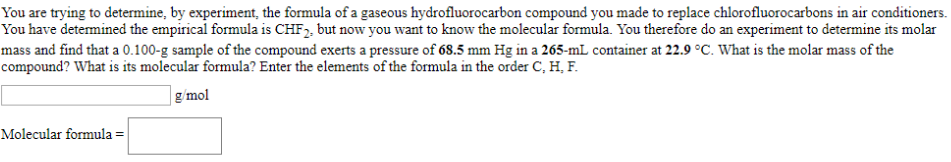# You are trying to determine, by experiment, the formula of a gaseous hydroflurocarbon compound you made to replace chlorofluorocarbons in air conditioners. You have determined the empirical formula is CHF2, but now you want to know the molecular formula. You therefore do an experiment to determine its molar mass and find that a 0.100-g sample of the compound exerts a pressure of 68.5 mm Hg in a 265-mL container at 22.9°C. What is the molar mass of the compound? What is its molecular formula? Enter the elements of the formula in the order C, H, F. _____ g/mol Molecular formula = _____